# Describing Supply and Demand Elasticities Sample Assignment

Essay Questions

1. Define the price elasticity of demand and the price elasticity of supply. What is the difference between elastic and inelastic demand?

The price elasticity of demand is a measure of the responsiveness of quantity demanded to changes in price. Specifically, the price elasticity of demand is the percentage change in quantity demanded divided by the percentage change in price. The price elasticity of supply is a measure of the responsiveness of quantity supplied to changes in price. Specifically, the price elasticity of supply is the percentage change in quantity supplied divided by the percentage change in price.

The difference between elastic and inelastic demand relates to whether the percentage change in quantity is larger or smaller than the percentage change in price. Specifically, demand is elastic if the percentage change in quantity is greater than the percentage change in price. Demand is inelastic if the percentage change in quantity is less than the percentage change in price.

(LO1)

2. Both the slope of the demand curve and the elasticity of demand are measures of how consumers alter their quantities demanded in response to changes in price. How are the two concepts different from each other? Given a negatively sloped straight-line demand curve, how will slope and elasticity differ? Given a vertical or horizontal demand curve, how will they differ?

The slope of the demand curve relates absolute changes in price and quantity demanded. Elasticity is a ratio of percentage changes. Whereas the slope of the demand curve will depend on the units in which price and quantity are measured, elasticity is a pure number and is independent of the units in which price and quantity are measured.

If the demand curve is a straight line, its slope is constant. However, the elasticity of demand along a negatively sloped straight-line demand curve changes at every point. It increases as we move up on the demand curve (higher prices and lower quantities), and decreases as we move down (lower prices and higher quantities).

Finally in measuring slope, the change in price is in the numerator and the change in quantity is in the denominator. In the case of elasticity, the percentage change in quantity is in the numerator and the percentage change in price is in the denominator. Hence, if the demand curve is vertical (slope equals infinity), the elasticity of demand is zero (perfectly inelastic). If the demand curve is horizontal (slope equals zero), the elasticity of demand is infinite (perfectly elastic).

(LO2)

3. Five brief scenarios are presented below. Apply the correct elasticity term to each scenario and explain your answer.

1. John must have an appendectomy. Upon arriving at the hospital he is told that for this week only they are doing abdominal operations at half price. Even in the face of a 50 percent price cut, John insists that he wants only one operation.
2. The Dell Corporation announces that it is willing to sell as many model 3000 computers as are ordered at a fixed price of \$500 per unit.
3. Northwest Airlines increased all its fares by 25 percent. As a result the number of passengers declined by 25 percent and the total revenue of Northwest Airlines remained the same.
4. Newsweek magazine raised its price to annual subscribers by 50 percent. As a result annual subscriptions fell by 30 percent. Although it lost some readership, Newsweek significantly increased its revenues.
5. The price of soybeans went up by 60 percent. In response farmers planted more soybeans and output increase by 70 percent.

1. John had a perfectly inelastic demand for abdominal surgeries. No matter what the percentage change in price, the percentage change in the quantity demanded was zero.
2. The Dell Corporation had a perfectly elastic supply curve of model 3000 computers. No matter what the percentage change in the quantity it supplied, the percentage change in price was zero.
3. Northwest Airlines had a unit elastic demand for its flights because the percentage gain in price was just offset by the percentage decline in quantity demanded.
4. Newsweek magazine had an inelastic demand for annual subscriptions because the percentage loss in subscribers was less than the percentage gain in price.
5. The supply of soybeans was elastic because the percentage increase in output was greater than the percentage increase in price.

(LO3)

4. Why is substitution the key factor in determining how elastic or inelastic a demand curve will be? Under what conditions will demand be more elastic?

Substitution is the key factor in determining how elastic or inelastic a demand curve will be. Buyers are more sensitive to changes in price when there are more goods that are available as substitutes. Therefore, the more substitutes, the more elastic the demand curve. If few substitutes are available, buyers will be less sensitive to changes in price and demand will be inelastic.

If you have more time to shop around, demand will be more elastic. The more specifically you define the product that you wish to buy, the more substitutes are available and the more elastic the demand is likely to be. The larger the portion of your budget that you devote to a product, the more elastic will be your demand. The more you think of your purchase as a luxury, the more elastic will be your demand.

(LO4)

5. What will happen to total revenue of a firm if it raises its price and its demand is elastic (or inelastic)?

If demand is elastic, the percentage change in quantity demanded is greater than the percentage change in price. Thus with a price increase, the quantity demanded drops by a greater percentage than price rises, so total revenue (price times quantity) goes down.

If demand is inelastic, this means the percentage change in quantity demanded is less than the percentage change in price. Thus with a price decrease, the quantity demanded increases by a smaller percentage than price drops, so total revenue (price times quantity) goes down.

(LO5)

6. What is the income elasticity of demand? How can it be used to determine whether a good is a normal good or an inferior good? What is the cross-price elasticity of demand? How can it be used to determine whether two goods are substitutes or compliments?

The income elasticity of demand measures the responsiveness of demand to a change in income. Specifically the income elasticity of demand is the percentage change in demand divided by the percentage change in income. Thus, if the income elasticity of demand is greater than zero, the good is a normal good—demand and income change in the same direction. If the income elasticity of demand is negative, the good is an inferior good—demand and income change in opposite directions.

The cross-price elasticity of demand measures the responsiveness of demand to a change in the price of related good. Specifically the cross-price elasticity of demand is the percentage change in demand for one good divided by the percentage change in the price of a related good. Thus, if the cross price elasticity of demand for two goods is greater than zero, they are substitutes. An increase the price of one results in an increase in the demand for the other (e.g. hot dogs and hamburgers). If the cross price elasticity of demand for the two goods is negative, they are compliments. An increase the price for one good results in a decrease in the demand for the other (e.g. hot dogs and mustard).

(LO6)

7. You started a pajama business four years ago and it is now becoming quite successful. You are thinking of hiring an economic consultant to help you rise to the next level in your industry. One consultant is familiar with the concept of elasticity; one is not. Which one would you hire? Explain your reasoning.

You should hire the consultant who is familiar with the concept of elasticity. Among many other things, you will want to know whether you should raise the price of your pajamas, lower them or keep the prices the same. A consultant familiar with the concept of elasticity can answer that question for you. If the demand for your pajamas is elastic, raising prices will lower your total revenues. If the demand is unit elastic, raising your price will not affect your total revenues. If demand is inelastic, you can increase your total revenues by raising the price of your pajamas. Furthermore you will be selling fewer pajamas and so your costs will fall even as your revenues are rising. Once you understand elasticity, supply and demand analysis becomes much more useful.

(LO7)

8. Define the price elasticity of demand.

The price elasticity of demand is a measure of the responsiveness of quantity demanded to changes in price. Specifically, the price elasticity of demand is the percentage change in quantity demanded divided by the percentage change in price.

9. Define the price elasticity of supply.

The price elasticity of supply is a measure of the responsiveness of quantity supplied to changes in price. Specifically, the price elasticity of supply is the percentage change in quantity supplied divided by the percentage change in price.

10. What is the difference between elastic and inelastic demand and supply?

The difference relates to whether the percentage change in quantity is larger or smaller than the percentage change in price. Specifically:

Demand or supply is elastic if the percentage change in quantity is greater than the percentage change in price.

Demand or supply is inelastic if the percentage change in quantity is less than the percentage change in price.

11. What is the key factor in determining how elastic or inelastic a demand curve will be? Explain.

The key factor in determining how elastic or inelastic a demand curve will be is substitution. The more goods are available as substitutes the more sensitive to changes in price buyers will be, and the more elastic the demand curve. If there are few substitutes available buyers will be less sensitive to changes in price and demand will be inelastic.

12. Under which of the following two scenarios would demand for hot dogs be more elastic? Explain.

1. You are at a baseball game at Wrigley Field and want to eat a hot dog.
2. It's Tuesday, you are planning the menu for a picnic you are going to have on Saturday and you decide to serve hot dogs.

Demand for hot dogs will be more elastic in scenario (b) because you have much more time to shop around for hot dogs. At the game if you want a hot dog, you've got to pay the price. The general lesson is the more time you have to shop the more elastic the demand.

13. Under which of the following two scenarios would demand be more elastic? Explain.

1. Demand for a camera.
2. Demand for a 35 mm SLR camera with auto rewind, advance, zoom, and flash.

Demand for a specific type camera is more elastic because many types of cameras take acceptable pictures. The general lesson is that the more specifically you define your purchase the more substitutes there are available and the more elastic the demand is likely to be.

14. Under which of the following two scenarios would demand be more elastic? Explain.

1. Demand for a new car.
2. Demand for a tank of gas for your current car.

Demand would be more elastic in the first case. Cars are much more expensive than a tank of gas so you are much more likely to shop around until you get the best price for a car than you are to drive all over town to save a few cents on a tank of gas. The general rule is the elasticity of demand for a certain product increases as the percentage of your budget spent on that product increases.

15. Under which of the following two scenarios would demand be more elastic? Explain.

1. Demand for blood pressure medicine by those with high blood pressure.
2. Demand for Clairol hair coloring.

Demand will be more elastic in the second case. There are several alternatives to the purchase of Clairol hair coloring but if one has high blood pressure one needs to take his/her medicine. The general lesson is the less the good is a necessity the more elastic is its demand curve.

16. How is the elasticity of supply related to time?

The longer the time period, the more elastic the supply. This is due to the increased production possibilities as time progresses.

17. Are the elasticities of demand and supply the same as the slopes of demand and supply curves? Explain.

No, they are not the same. If a demand curve is a straight line, its slope is constant. However, the elasticity of demand along a straight-line demand curve is not constant. It increases as we move up on the demand curve (higher prices and lower quantities), and decreases as we move down (lower prices and higher quantities). If the demand curve is vertical (slope equals infinity), the elasticity of demand is zero. If the demand curve is horizontal (slope equals zero), the elasticity of demand equals to infinity.

In the case of the supply curve, the relationship between the slope and elasticity is slightly different. If the supply curve intercepts the quantity axis, elasticity of supply rises as we move up on the line (higher prices and higher quantities). If the line intercepts the price axis, elasticity of supply decreases as we move up on the line.

18. What happens to total revenue if a firm raises its price and its demand is elastic?

If demand is elastic, the percentage change in quantity demanded is greater than the percentage change in price. Thus with a price increase the quantity demanded drops by a greater percentage than price rises, so total revenue (price times quantity) goes down.

19. What happens to total revenue if a firm lowers its price and its demand is inelastic?

If demand is inelastic, the percentage change in quantity demanded is less than the percentage change in price. Thus with a price decrease the quantity demanded increases by a smaller percentage than price drops, so total revenue (price times quantity) goes down.

20. What is the income elasticity of demand?

The income elasticity of demand measures the responsiveness of demand to a change in income. Specifically the income elasticity of demand is the percentage change in demand divided by the percentage change in income.

21. How can the income elasticity of demand be used to determine whether a good is a normal good or an inferior good?

The income elasticity of demand is the percentage change in demand divided by the percentage change in income. Thus, if? the income elasticity of demand is greater than zero, the good is a normal good—demand and income change in the same direction. If the income elasticity of demand is negative, the good is an inferior good—demand and income change in opposite directions.

22. What is the cross-price elasticity of demand?

The cross-price elasticity of demand measures the responsiveness of demand to a change in the price of another (related) good. Specifically the cross-price elasticity of demand is the percentage change in demand divided by the percentage change in the price of another good.

23. What are substitute goods? Give an example.

Substitute goods are goods that can be used in place of one another. An example is a pencil and a pen.

24. What are complementary goods? Give an example.

Complementary goods or complements are goods that are used in conjunction with other goods. An example is a baseball glove and a baseball.

25. How can the cross-price elasticity be used to determine whether two goods are complements or substitutes?

The cross-price elasticity is the percentage change in demand for one good divided by the percentage change in the price of a related good. If the value of the cross-price elasticity is positive, the goods are substitutes—the change in the price of one good and the change in the demand for the related good are in the same direction. If the value is negative, the goods are complements—the change in the price of one good and the change in the demand for the related good change in opposite directions.

26. If two goods have a cross-price elasticity value of +3, are they substitutes or complements?

When a cross-price elasticity is positive it means that an increase in the price of one good will cause an increase in the demand for another good. This indicates that the two goods are substitutes.

27. Suppose that the price elasticity of demand is 6. If price rises by 10% how much will quantity demanded fall?

A price elasticity of demand of 6 means that for every one percent increase in price the quantity demanded will fall by 6%. Since here price rises by 10%, quantity demanded will fall by 60%.

28. Suppose that the price elasticity of supply is 0.5. If price falls by 10% how much will quantity supplied fall?

A price elasticity of supply of 0.5 means that for every one percent fall in price the quantity supplied will fall by 1/2%. Since here price falls by 10%, quantity supplied will fall by 5%.

29. Bill's Homemade Pizza recently increased the price of its super jumbo size pepperoni pizza by 12%. As a result of this price increase the quantity demanded for this type of pizza decreased by 3%. What is the price elasticity of demand for Bill's super jumbo size pepperoni pizza?

E = 3/12 = .25

Problems and Applications

30. Consider the following demand curve: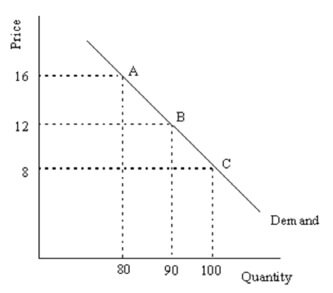1. Calculate the elasticity of demand between points A and B.
2. Calculate the elasticity of demand between points B and C.

(a) The elasticity of demand between points A and B:

Percentage change in quantity = (10/85) × 100 = 11.8

Percentage change in price = (4/14) × 100 = 28.6

E = (11.8)/(28.6) = 0.41

(b) The elasticity of demand between points B and C:

Percentage change in quantity = (10/95) × 100 = 10.5

Percentage change in price = (4/10) × 100 = 40

E = (10.5)/(40) = 0.26

31. Consider the following supply curve: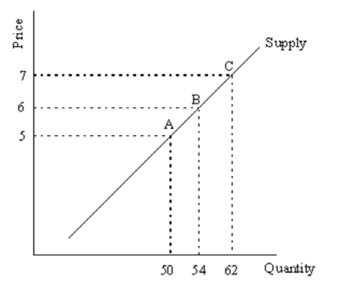1. Calculate the elasticity of supply between points A and B.
2. Calculate the elasticity of supply between points B and C.

(a) The elasticity of supply between points A and B:

Percentage change in quantity = (4/52) × 100 = 7.7

Percentage change in price = (1/5.5) × 100 = 18.2

E = (7.7)/(18.2) = 0.42

(b) The elasticity of supply between points B and C:

Percentage change in quantity = (8/58) × 100 = 13.8

Percentage change in price = (1/6.5) × 100 = 15.4

E = (13.8)/(15.4) = 0.9

32. Use the following demand curve to show that elasticity is not the same as slope.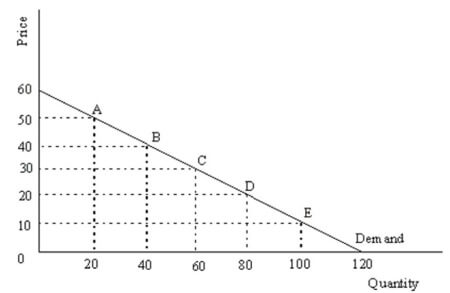To see that elasticity and slope are different it is sufficient to show that while the slope is constant at all points on the demand curve elasticity is not. Since the demand curve is linear it has a constant slope, the slope of the demand curve above is –½. Elasticity varies at all points along the demand curve. For example compute the point elasticity for points B and C.

Elasticity at point B:

percentage change in quantity = [(60 – 20)/40] × 100 = 1

percentage change in price = [(50 – 30)/40] × 100 = 1/2

elasticity = 1/(1/2) = 2.0

Elasticity at point C:

percentage change in quantity = [(80 – 40)/60] × 100 = 2/3

percentage change in price = [(40 – 20)/30] × 100 = 2/3

elasticity = (2/3)/(2/3) = 1.0

Since elasticity is different at points B and C but slope is the same we can see that slope and elasticity are not the same.

33. Demonstrate graphically and explain verbally a perfectly elastic demand curve.

A perfectly elastic demand curve is one for which consumers are so sensitive to price changes that any price change results in quantity demanded dropping to zero. In terms of the diagram, a perfectly elastic demand curve is a horizontal line, as shown below: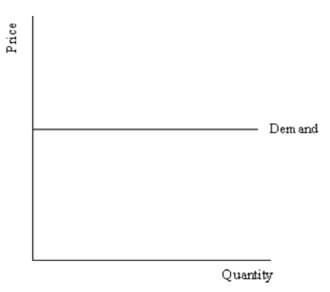34. Demonstrate graphically and explain verbally a perfectly inelastic demand curve.

A perfectly inelastic demand curve is one for which consumers are so insensitive to price changes that they will pay any price to get the quantity they demand. In terms of the diagram, a perfectly inelastic demand curve is a vertical line, as shown below: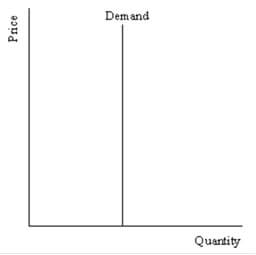35. Consider the following demand curve: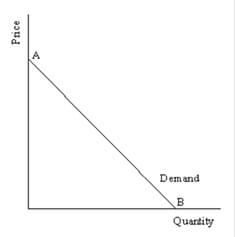What is the price elasticity at point A? At point B?

The price elasticity at point A is infinity; at point B it is zero.

36. Consider the following three diagrams: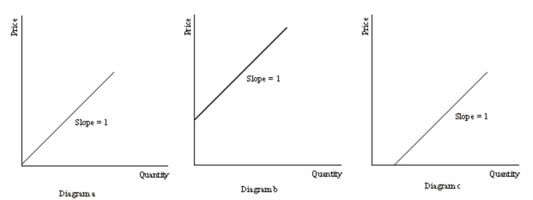1. Which of the diagrams above demonstrates a unit elastic supply curve? How can you tell?
2. Which of the diagrams above demonstrates an inelastic supply curve? How can you tell?

(a) Diagram (a) shows a unit elastic supply curve. This is the case because the supply curve originates at the origin.

(b) Diagram (c) shows an inelastic supply curve. This is the case because the supply curve originates from the horizontal axis.

37. Suppose you own two Domino's Pizza franchises in your town. After reading the latest issue of Pizza Monthly, you have concluded that both of your locations are generating below average revenue. You hire a local economics professor to conduct a pricing experiment. Here is her report:

Location 1: A 10% increase in price resulted in a 5% drop in the quantity demanded.

Location 2: A 10% increase in the price resulted in a 20% drop in the quantity demanded.

Using this information, how should you alter your pricing policy to increase your revenue at each location?

You should increase the price of your pizzas at location 1 and decrease the price at location 2. The reason for the difference is the elasticity of demand at each location. At location 1 E = 0.5 (demand is inelastic), thus an increase in price will bring about an increase in revenue. In contrast, at location 2 E = 2.0 (demand is elastic); a decrease in price will bring about an increase in revenue. Whether this increases profits, however, depends upon costs.

38. Consider the following demand curve for Starbucks coffee.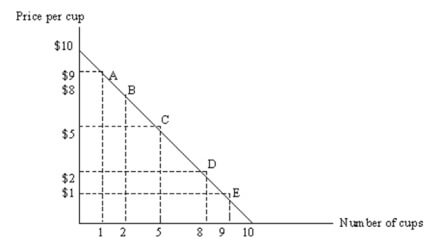1. Suppose the current price is \$8 per cup. Using elasticity of demand, explain why Starbucks should not increase the price in order to increase total revenue.
2. Suppose the current price is \$2 per cup. Using elasticity of demand, explain why Starbucks should not lower the price in order to increase total revenue.

(a) At this range on the demand curve, demand is elastic. Demand elasticity between points A and B is (1/1.5)/(1/8.5) = 5.67. If demand is elastic, Starbucks must lower the price in order to increase total revenue. Note that total revenue at \$8 a cup is \$16. If price increases to \$9 a cup, total revenue decreases to only \$9.

(b) At this range on the demand curve, demand is inelastic. Demand elasticity between points D and E is (1/8.5)/(1/1.5) = 0.17. If demand is inelastic, Starbucks must increase the price in order to increase total revenue. Note that total revenue at \$2 a cup is \$16. If price decreases to \$1 a cup, total revenue decreases to only \$9.

39. Demonstrate graphically and explain verbally the impact of an increase in income on the demand of a normal good.

Normal goods are goods that people buy more of when their income increases. In terms of a demand curve picture, this is illustrated as a rightward shift of demand. Note: The income elasticity of demand would measure the percentage change in demand from point A on D0 to point B on D1 divided by the percentage change in income.

The diagram: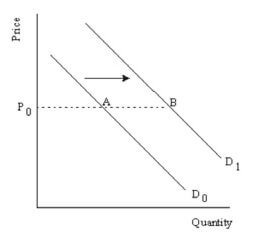40. Demonstrate graphically and explain verbally the impact on price and quantity when supply decreases and demand is highly elastic.

We can see that demand is highly elastic, as the demand curve is relatively flat. When supply decreases from S0 to S1 equilibrium changes from point A to point B. This results in a large drop in quantity (Q1 < Q0) and a small increase in price (P1 > P0).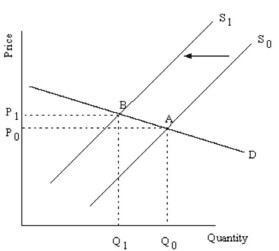41. The elasticity of demand for sunscreen is 0.8 and the elasticity of supply is 2.2. Suppose that the demand for sunscreen increased by 10% during an unseasonably hot month. Calculate the percentage change in the equilibrium price of sunscreen.